# How to solve a Square-1

A detailed walkthrough guide on how to solve a Square-1 (aka SQ-1) cube. We've tried hard to make this guide as easy to follow as possible. If you have an issues or recommendations please don't hesitate to reach out to us and let us know 😎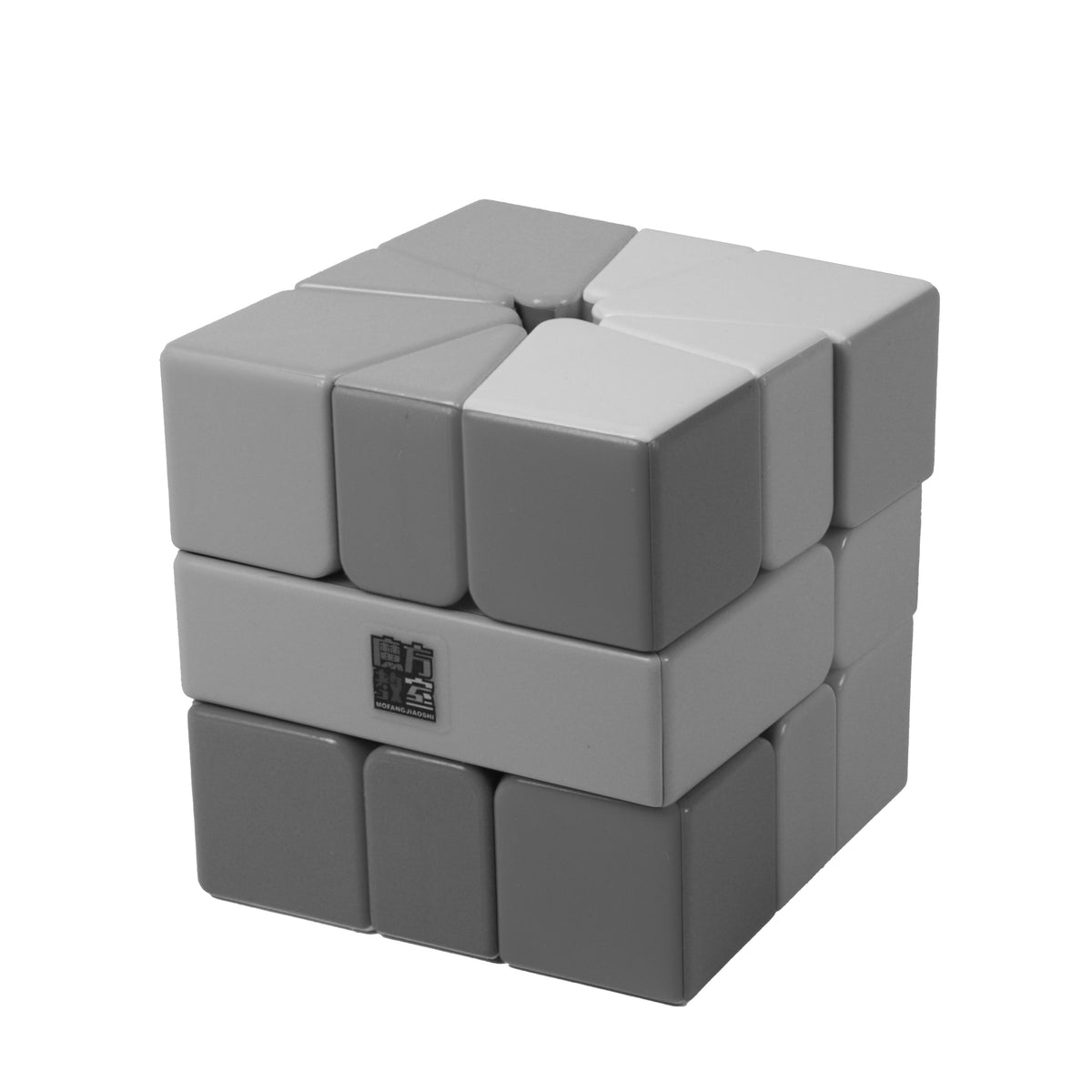### step 1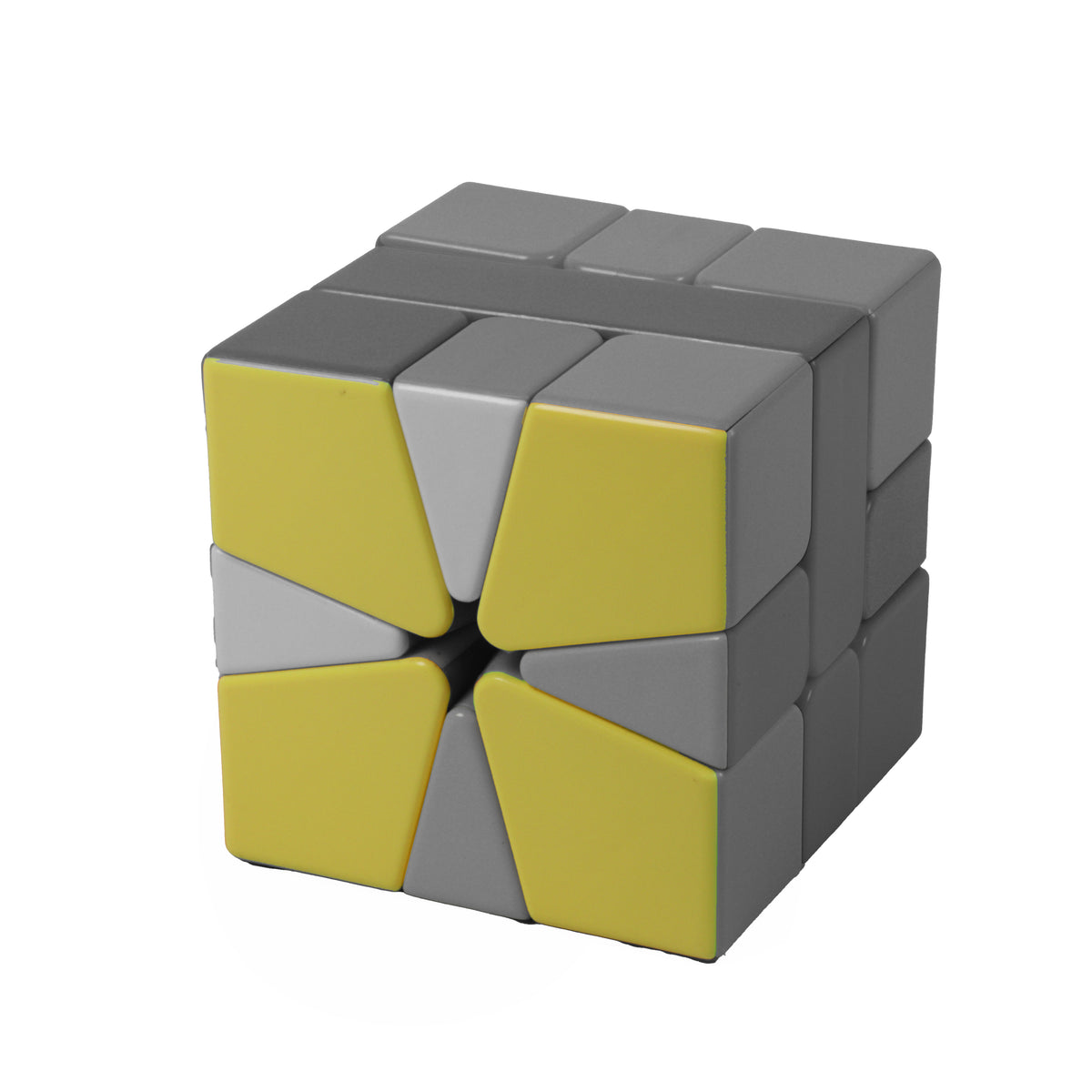### step 2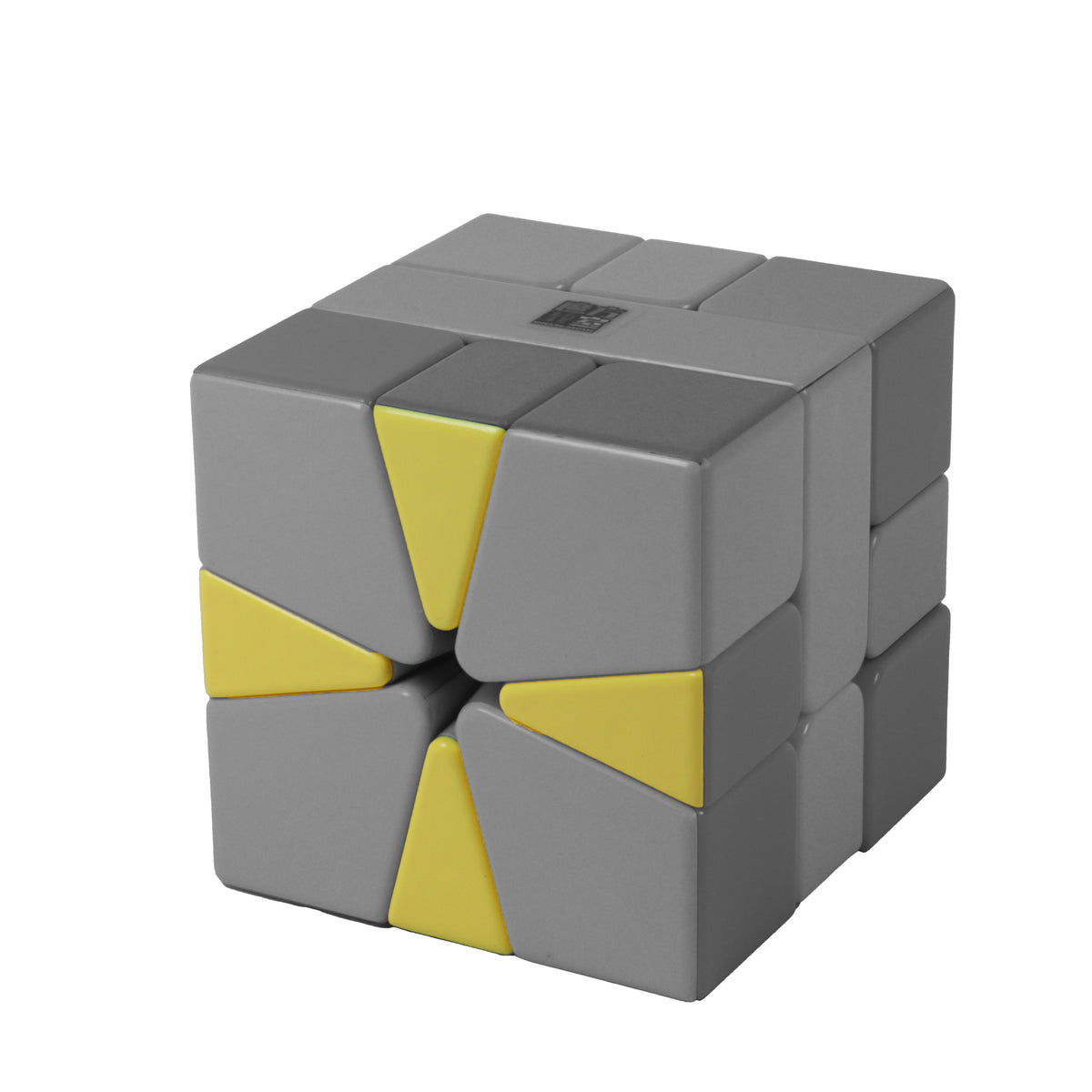### step 3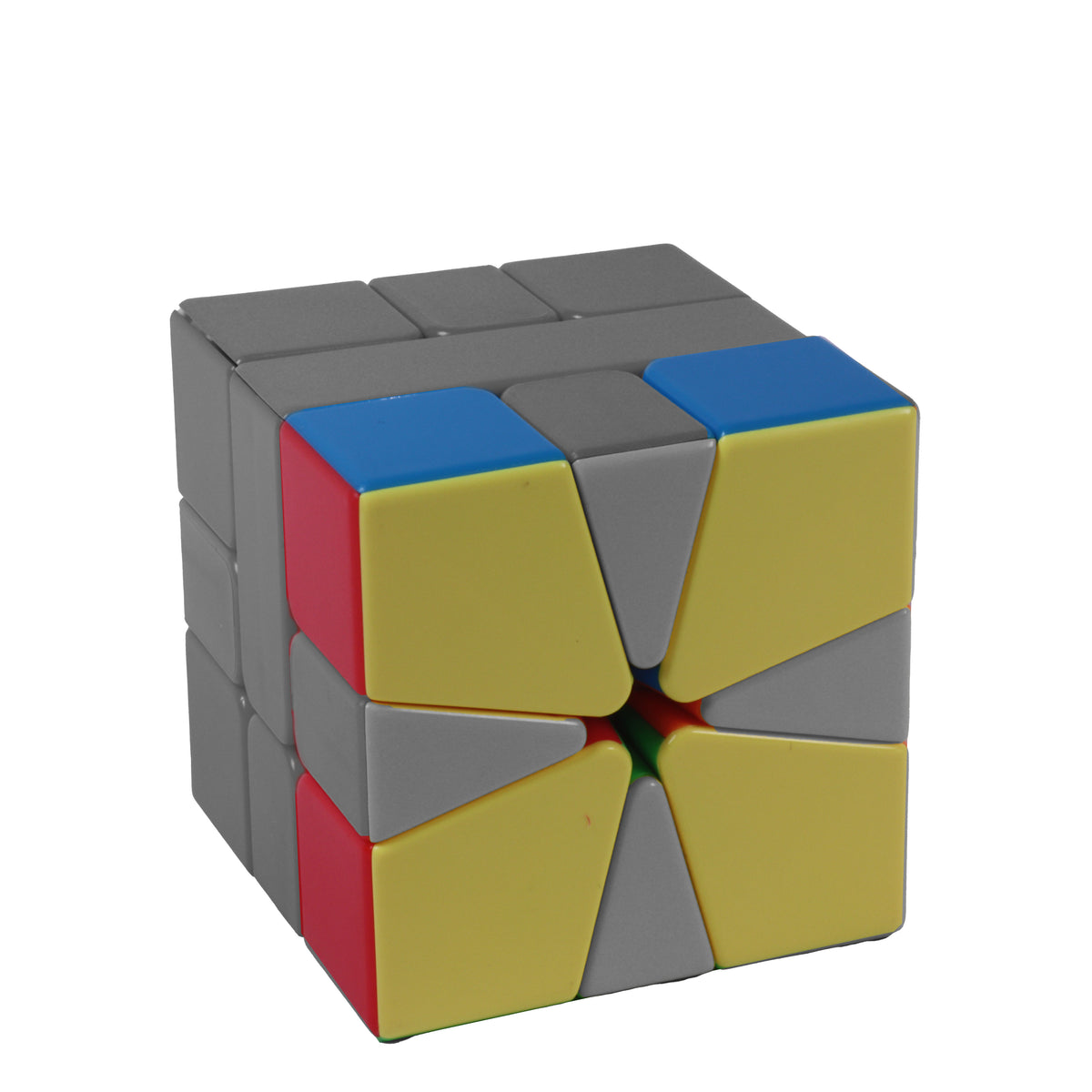### Notation

A typical Square-1 move looks something like this (1,0). That may look a little alien to you beginners but it's really simple to solve your Square-1 using these types of moves.

The first number in the bracket refers to the TOP layer of the Square-1 whilst the second number refers to the BOTTOM layer.
Think of it like this:
(top,bottom)

You will also come across a / in your journey of learning the Sq-1. This indicates a 180° rotation of the right hand side of the cube (as shown in the 3rd picture below).

Note: Each number inside the bracket is equal to a 30° rotation of that layer. The bigger corner pieces are equal to 60° and the smaller edges are 30°. This means that a full 90° rotation of the top or bottom layer would look like (3,0) or (0,3).

(1,0) - Turn top layer 30° clockwise (1 small piece)
(-1,0) - Turn top layer 30° anticlockwise
(0,1) - Turn bottom layer 30° anticlockwise
(0,-1) - Turn bottom layer 30° anticlockwise

A (2,0) or (4,0) would just mean you are turning the top layer 2 small pieces/1 large corner piece or 4 small pieces/2 corner pieces before you slice.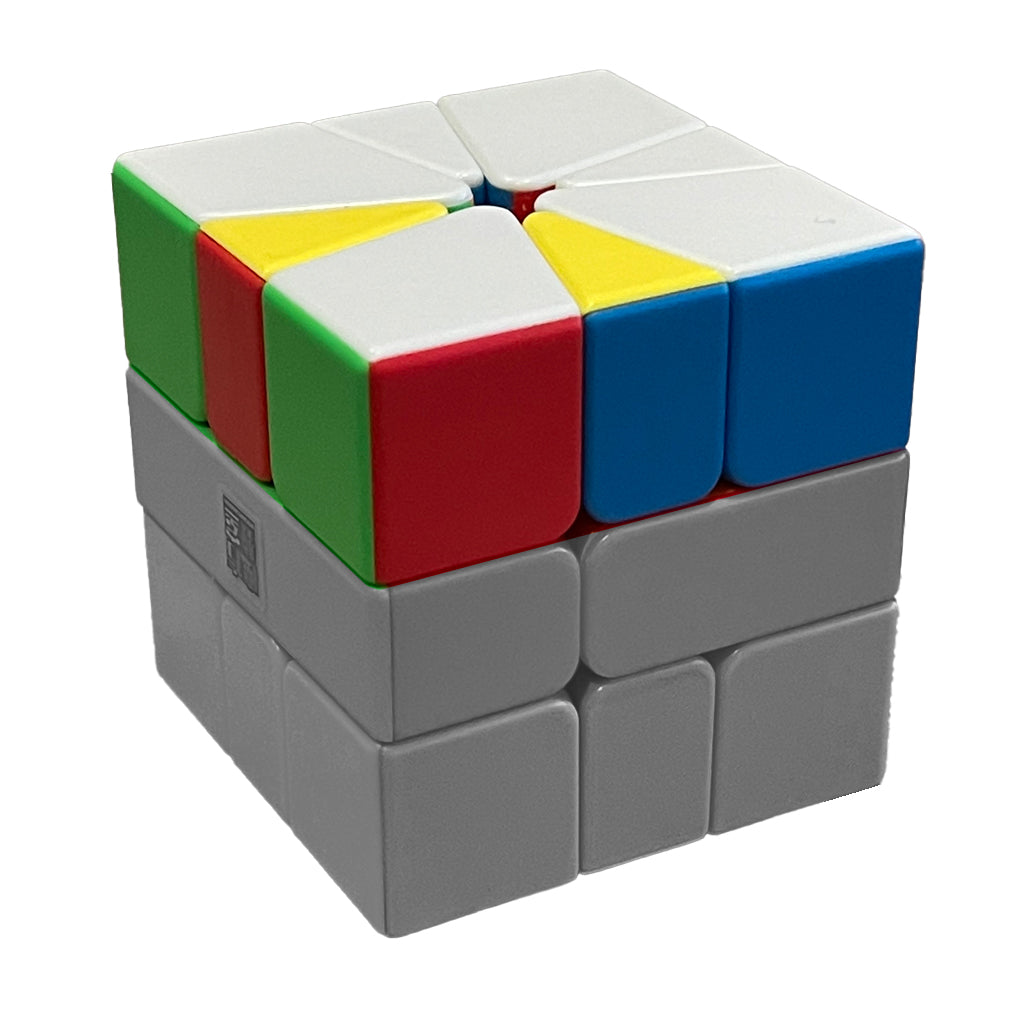### (1,0)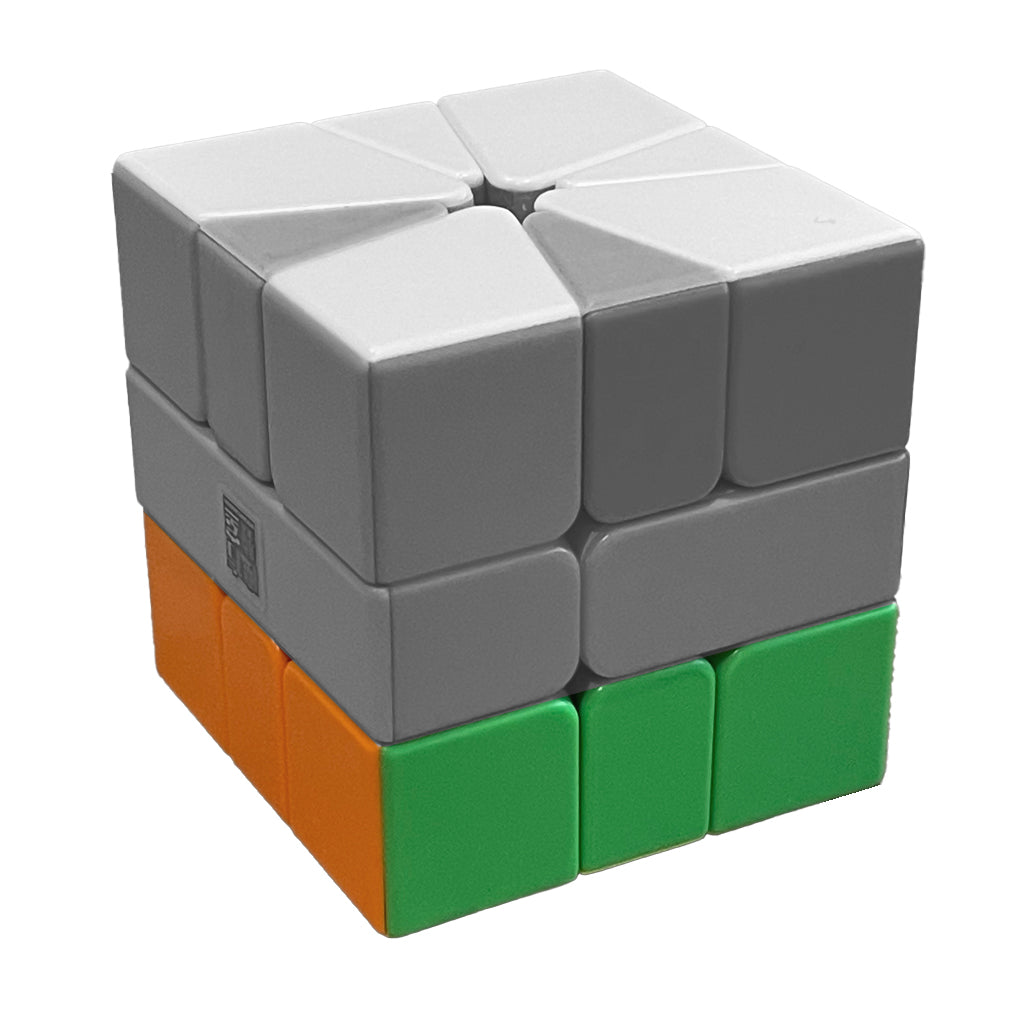### (0,1)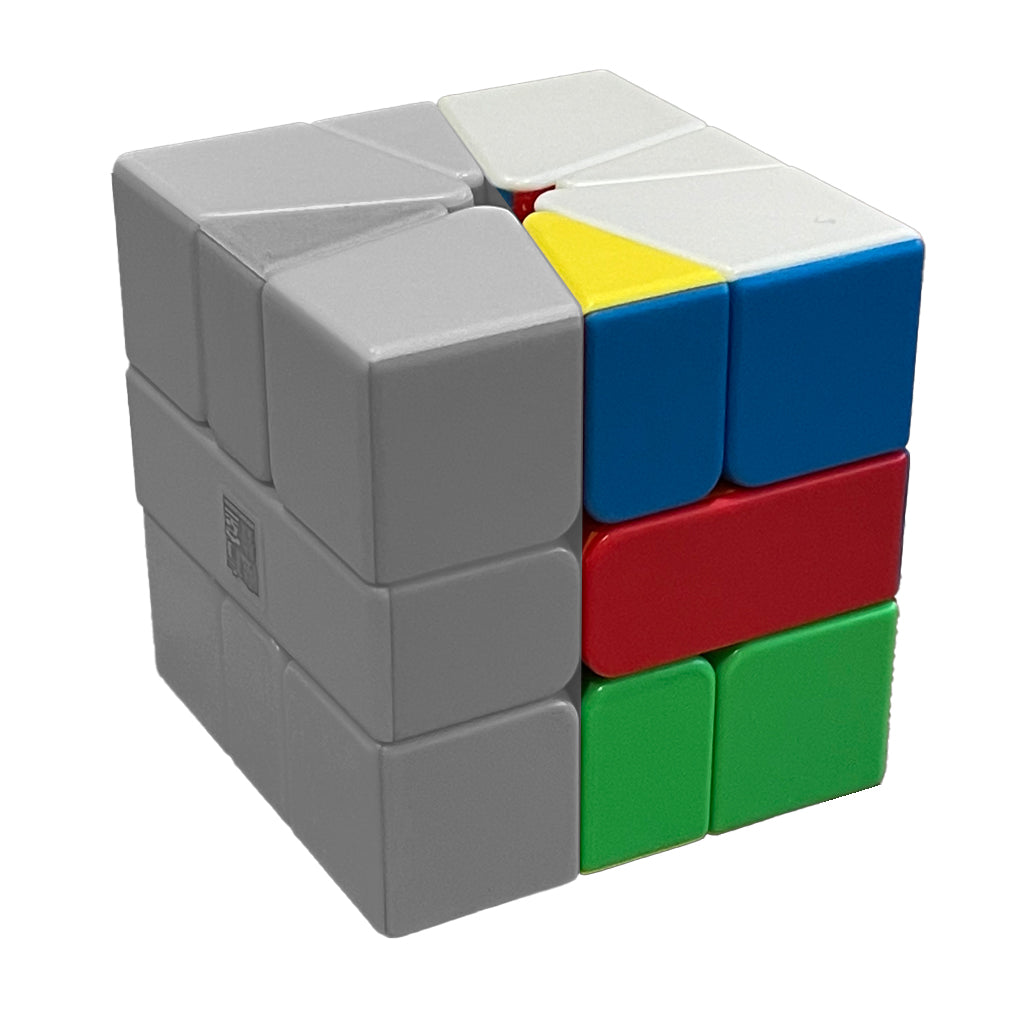### /

Example Algorithm: (1,0) / (3,0) / (-1,0) (video above) - In this move we turn the top layer 30° clockwise and do a slice by rotating the right side fo the cube 180° then we turn the top layer a full 90° and perform another slice before finishing off with a -30° of the top layer. If this confuses you just following along with the videos in each step to quickly get the hang of it.

### Getting a Cube

We don't mean you've got to go and get your cube, its obviously in your hand if you're reading this guide. In this step we are going to get our Square-1 back into a cube shape. If you already have a cube shape than PHEW! That was easy, wasn't it? 🤣 If not, sorry, you've got to battle on through.

Cube Shape infographic is coming soon.

### Step 1 - The 8 Corners

In step 1 we are going to move all of the corners to the correct layer. Using the middle bars as a guide, make sure your aware which colour should be at the top and bottom. In my case, white goes at the top and yellow goes at the bottom.

There are 2 basic algorithms you are going to need and it's as follows: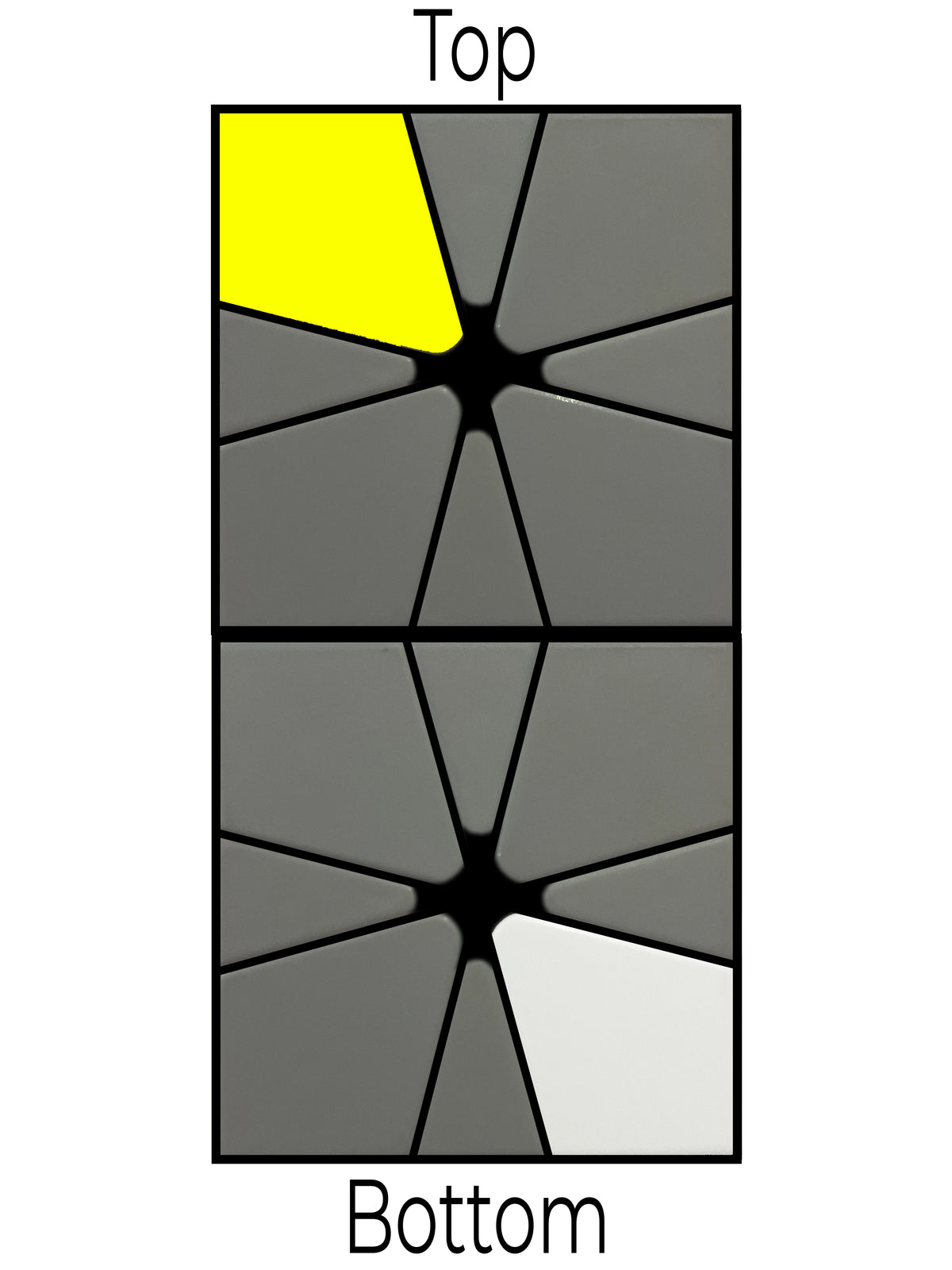Alg:      (1,0) / (3,0) / (-1,0)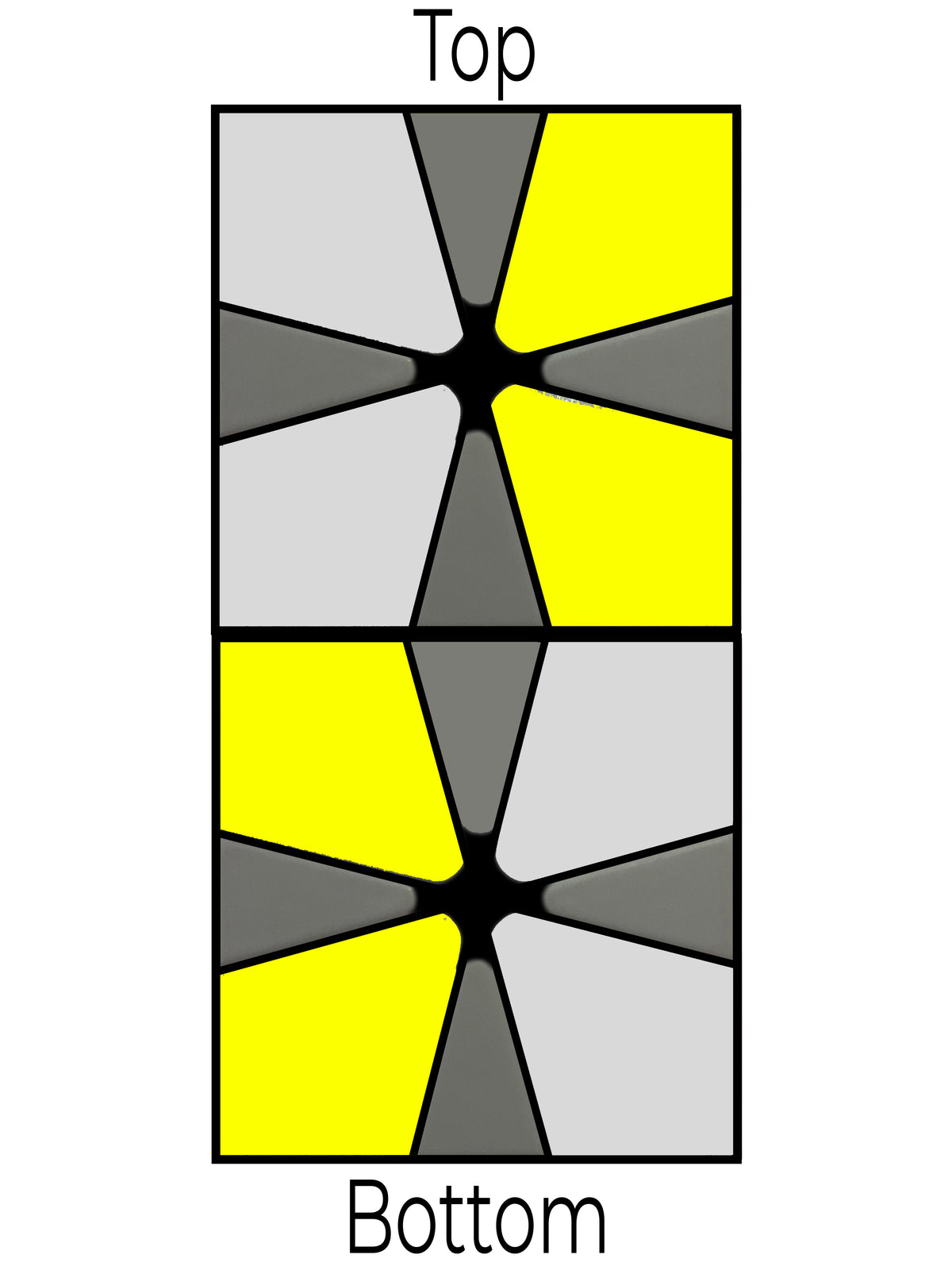Alg:      (1,0) / (-1,0)

### Step 2 - The 8 Edges

In step 2  we are going to bring the white edges to the top layer and the yellow edges to the bottom layer. Beware that this may be different for your cube, depending on brand and colour scheme etc....

We only need to know 2 algorithms here.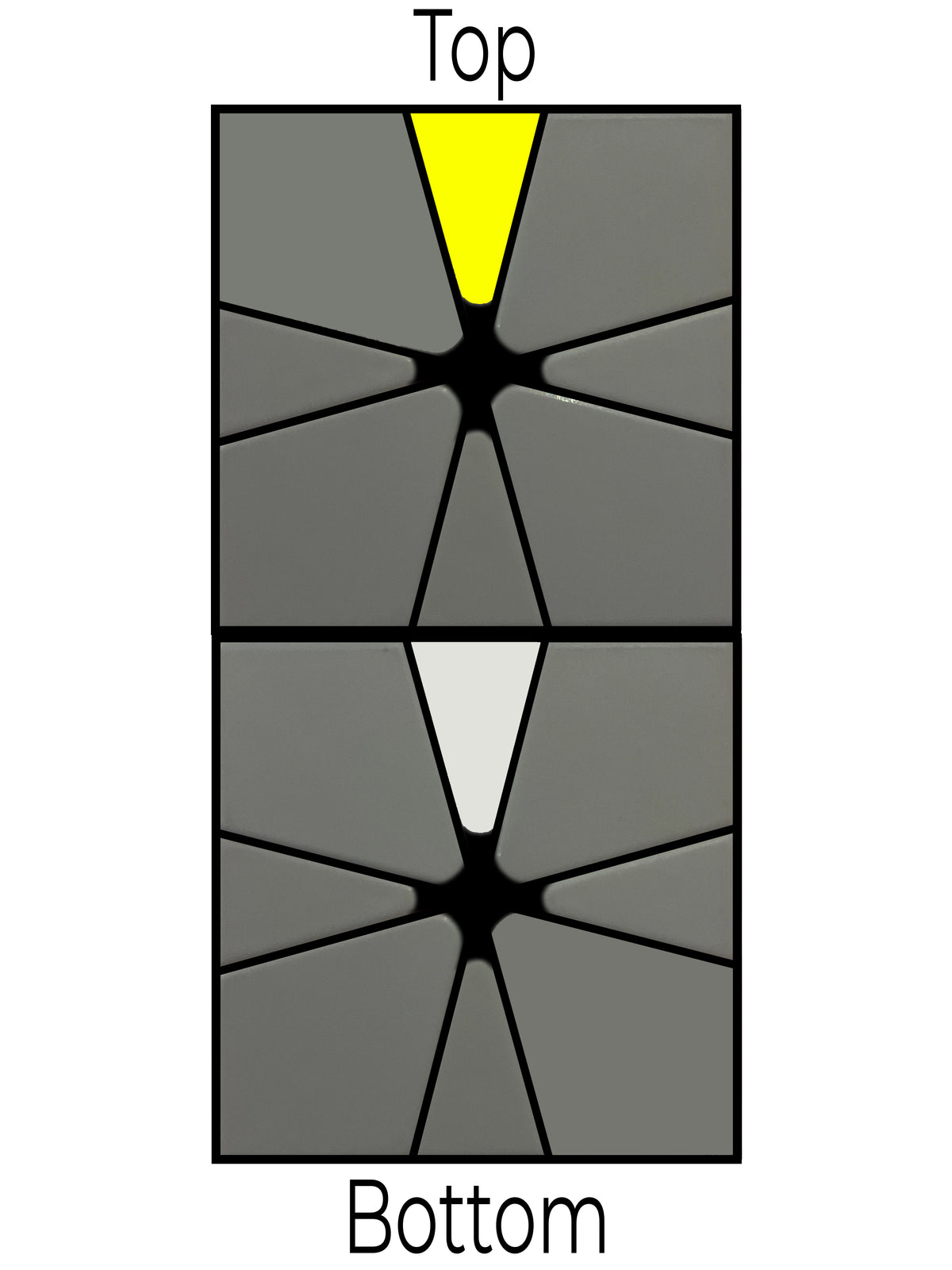Alg:      [(0,-1) / (-3,0) / (4,1)] / [(-4,-1) / (3,0) / (0,1)]

Tip: The last 3 moves in green are an exact reflection of the first 3 moves. This is a great way to remember it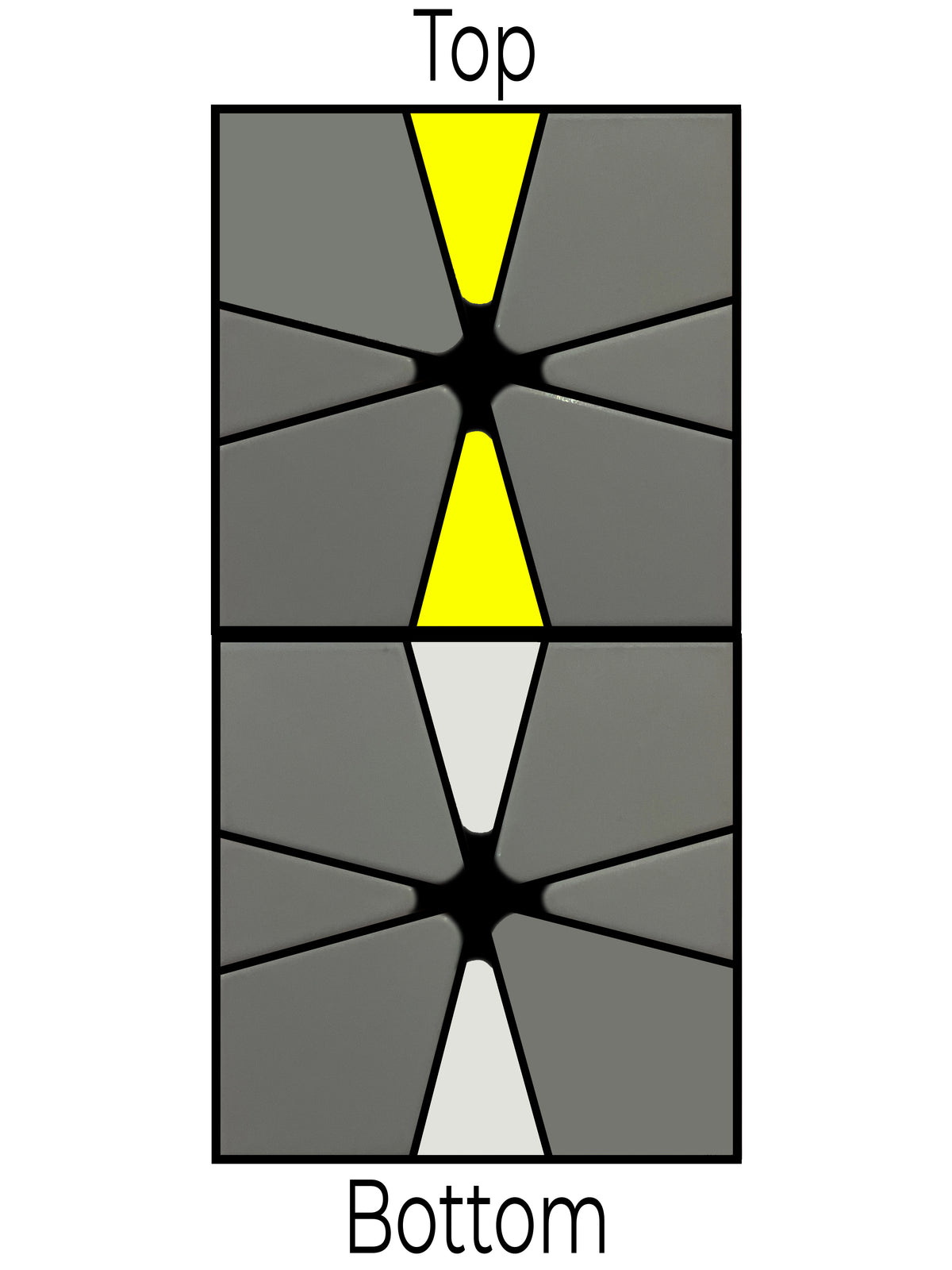Alg:      (0,-1) / (1,1) / (-1,0)

### Step 3 - Permuting the Corners

In step 3 we are going to move the corners around so that they are all in the correct place. Simply use the image below and the corresponding algorithm shown next to it to manoeuvre the corners around.

We are going to need to know a few algorithms in this step, but as long as your confident with the moves and notations then it'll be really easy. If not, watch the video and follow along 😃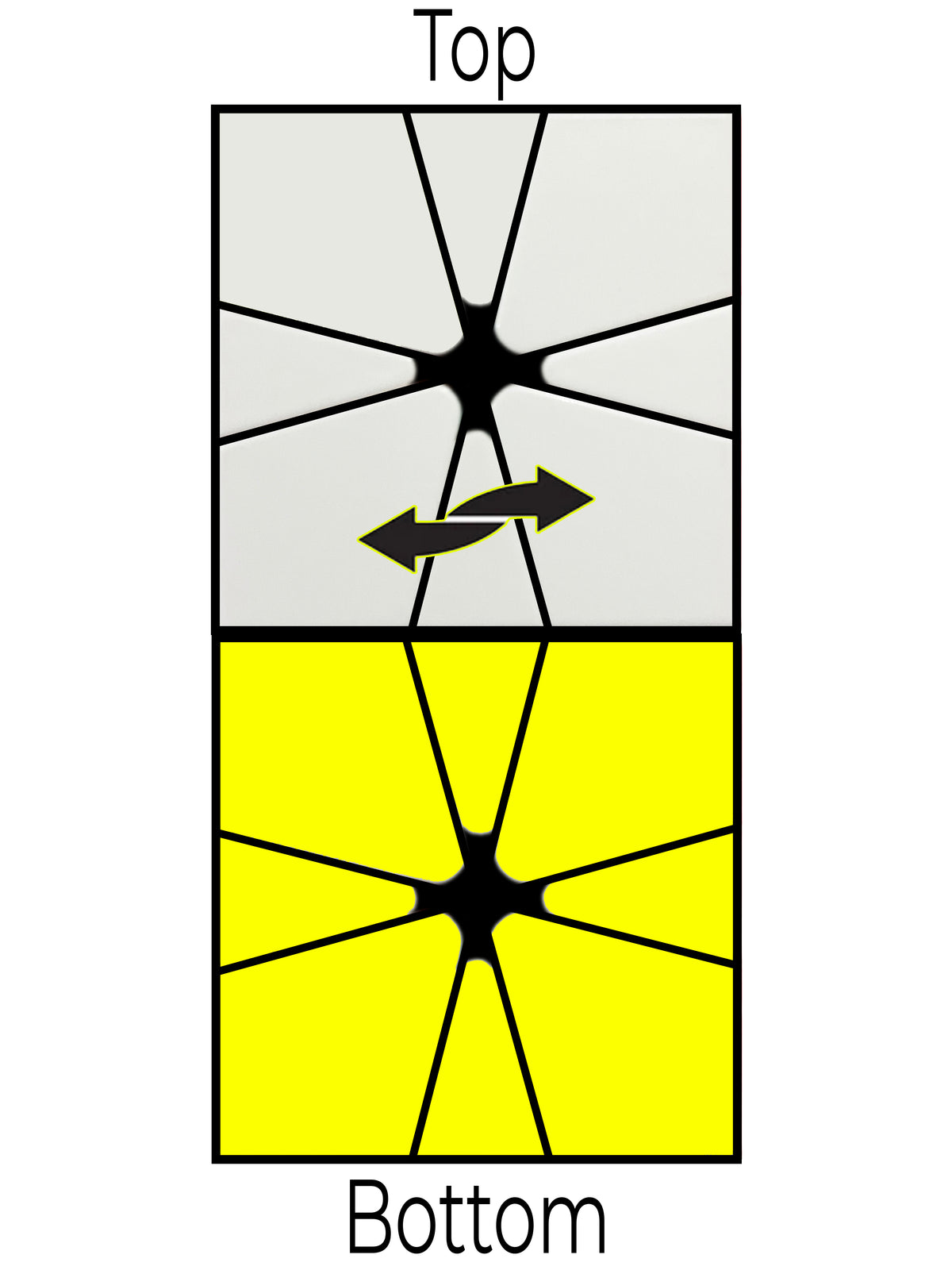Alg 1:       / (3,-3) / (3,0) / (-3,0) / (0,3) / (-3,0) /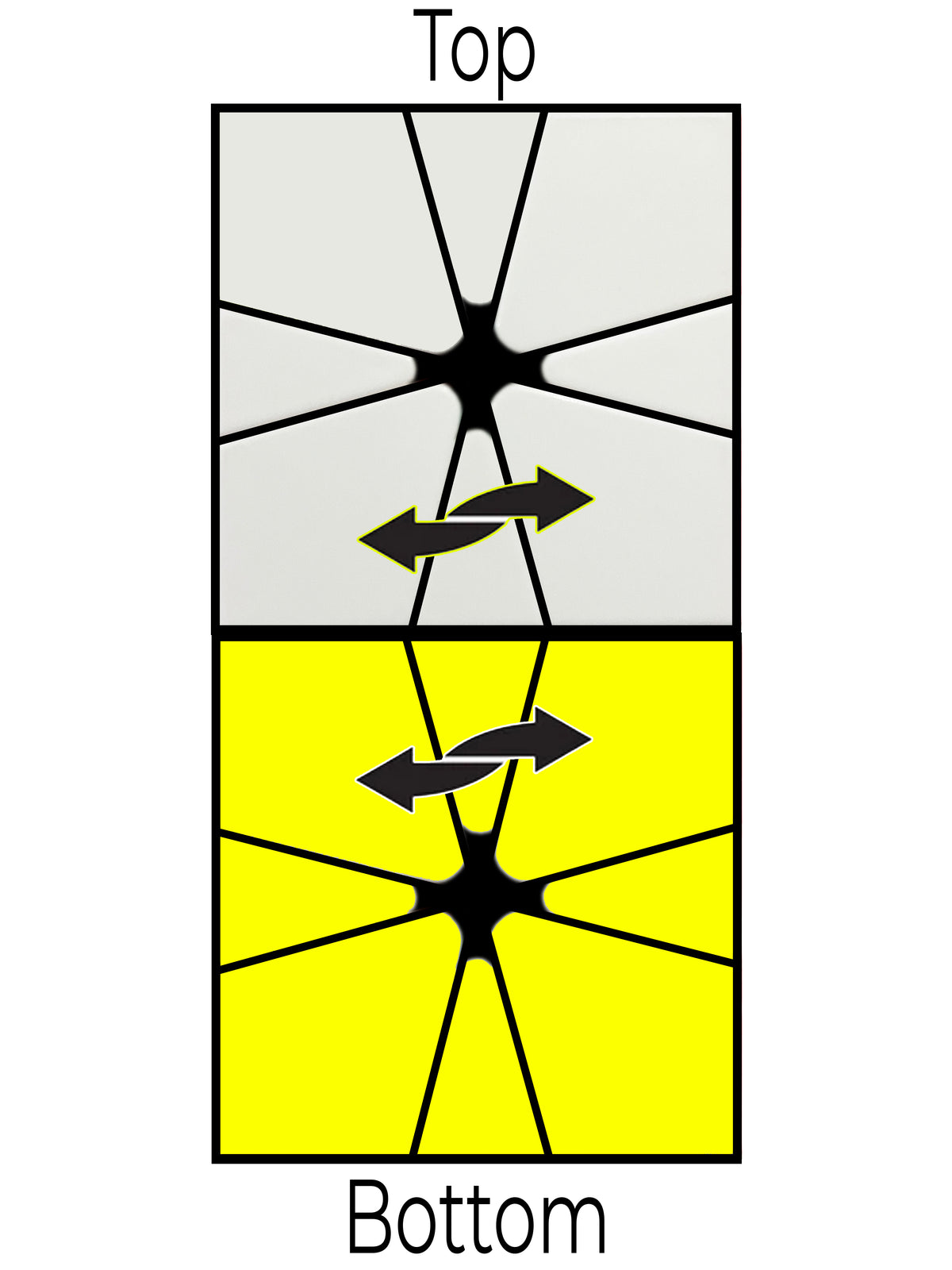Alg 2:      / (-3,0) / (3,3) / (0,-3) /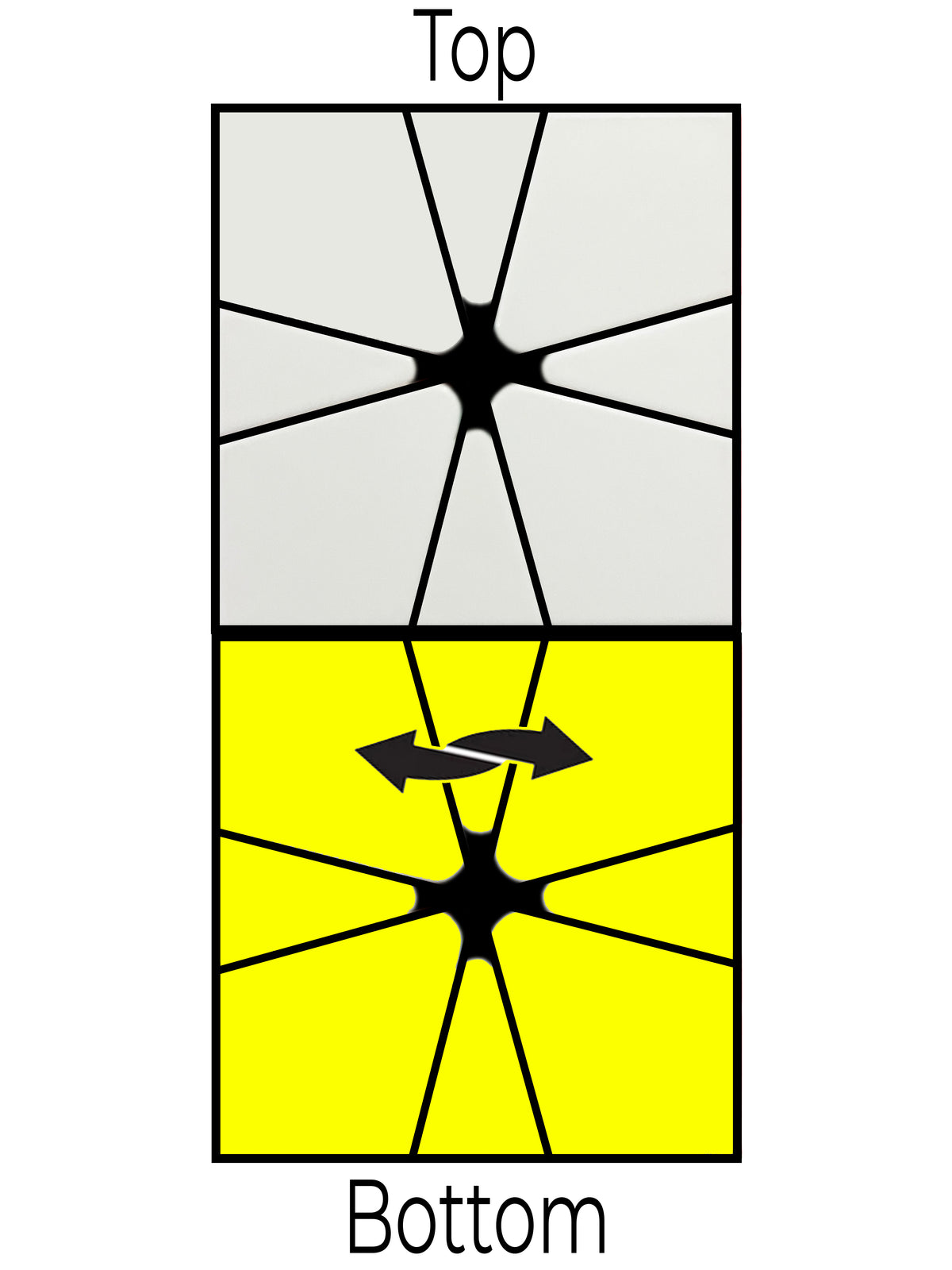Alg 3:      / (3,-3) / (0,3) / (-3,0) / (3,0) / (-3,0) /

Tip: The next 2 algorithms are almost identical, the part of the algorithm in blue is the only difference. This is an easy way to remember both the algorithms. If you are swapping the 2 corners on the bottom layer, the 2nd and 4th move require you to move the bottom layer, the opposite is true with alg 5, (where the 2nd and 4th move are the top layer).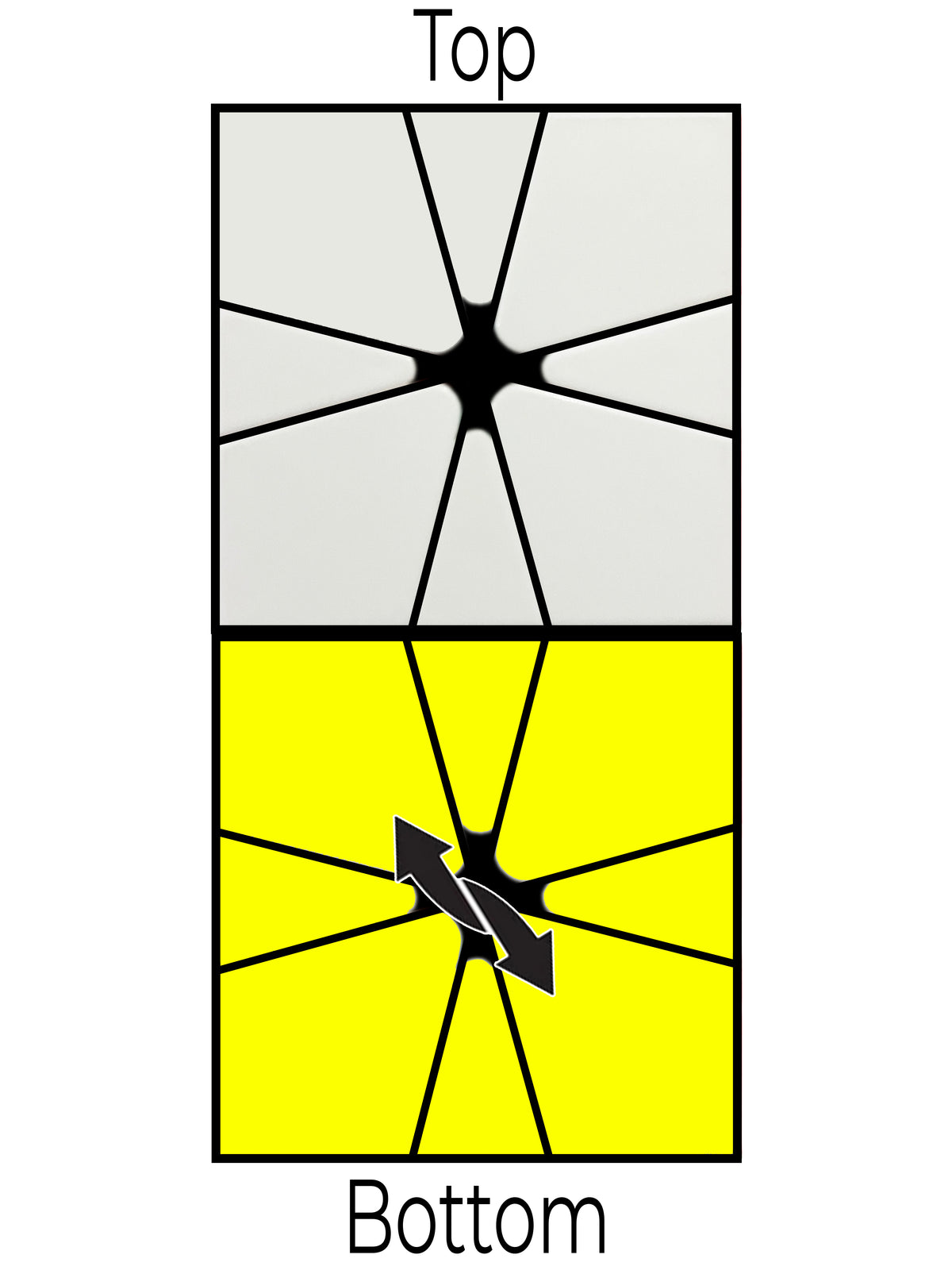Alg 4:      / (3,3) / (0,3) / (3,3) / (0,3) / (3,3) /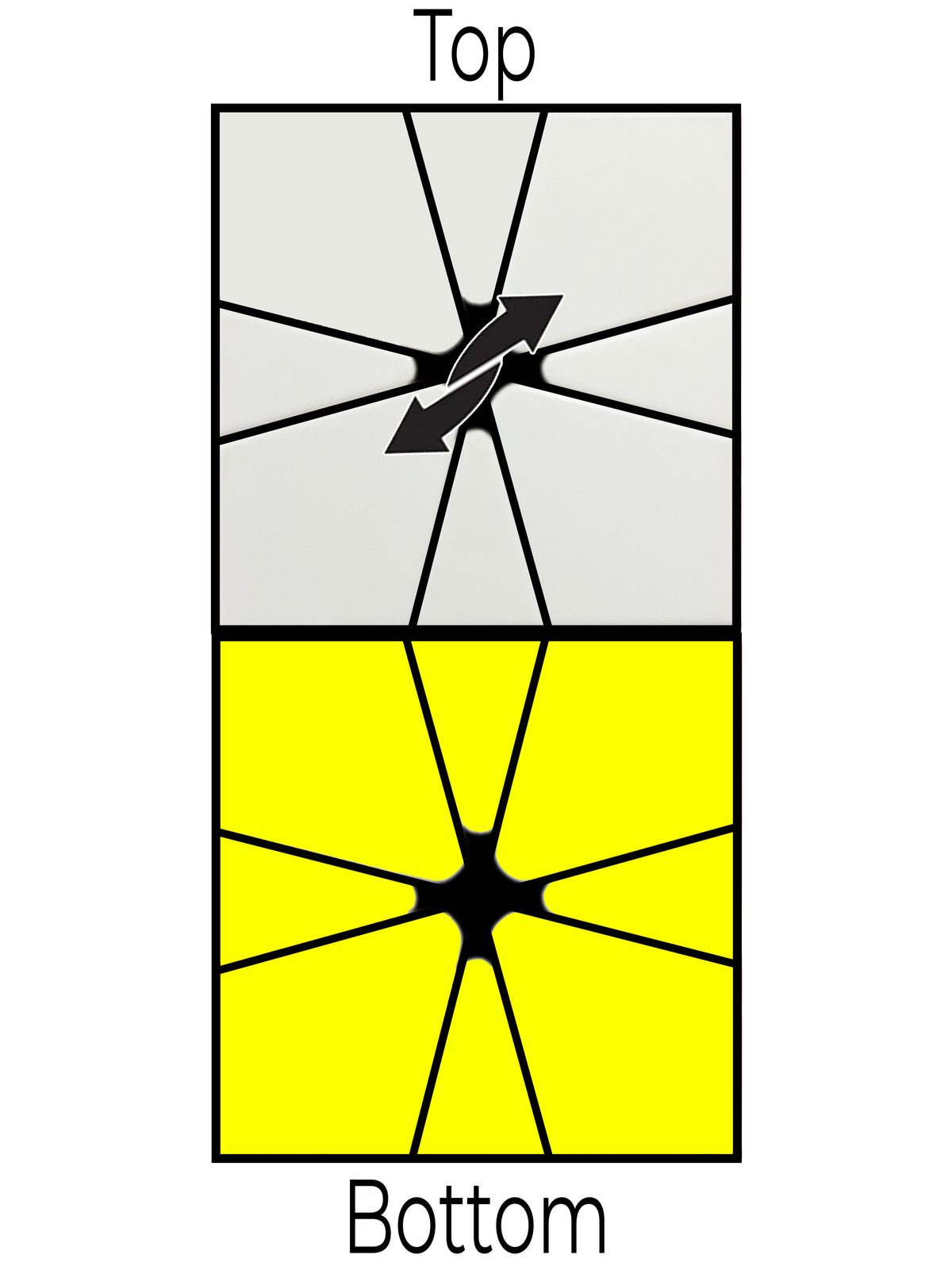Alg 5:      / (3,3) / (3,0) / (3,3) / (3,0) / (3,3) /

### Step 4 - Permuting the Edges

In step 4 we need to move the edges around. This is the last step before having a solved cube so let's crack on. Unfortunately there is a very long algorithm which we need to use. This may take some time to learn but it's well worth remembering if you're serious about learning to solve the SQ-1.

You may need to perform the algorithms multiple times and rotate the top/bottom layers

Algorithm 1 and 2 are also known as parity and are particularly long and difficult algs. Beware!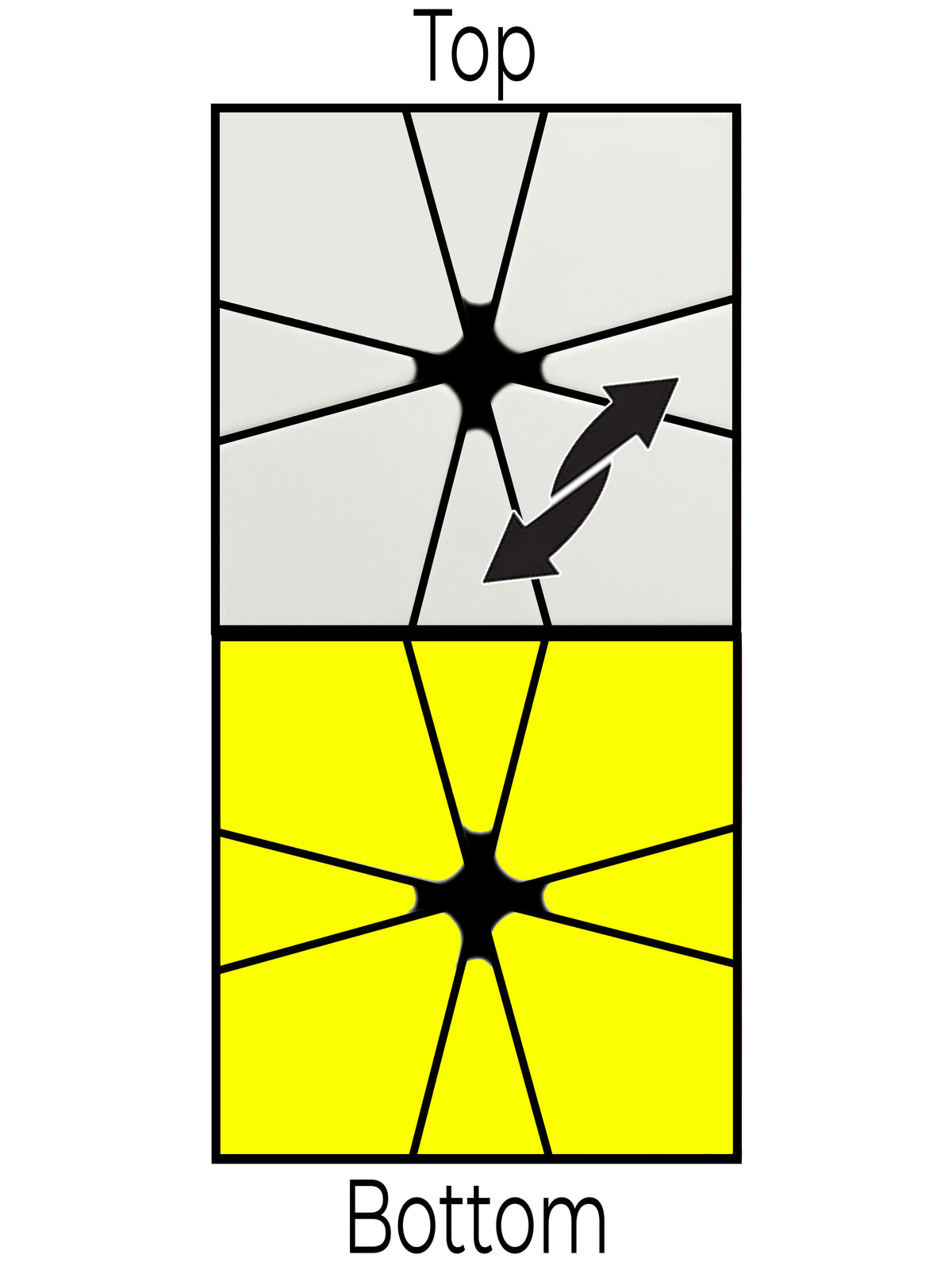Alg 1:       / (-3,0) / (0,3) / (0,-3) / (0,3) / (2,0) / (0,2) / (-2,0) / (4,0) / (0,-2) / (0,2) / (-1,4) / (0,-3) / (0,3)

Note: Be careful, this is quite a long alg. Take your time if you are a beginner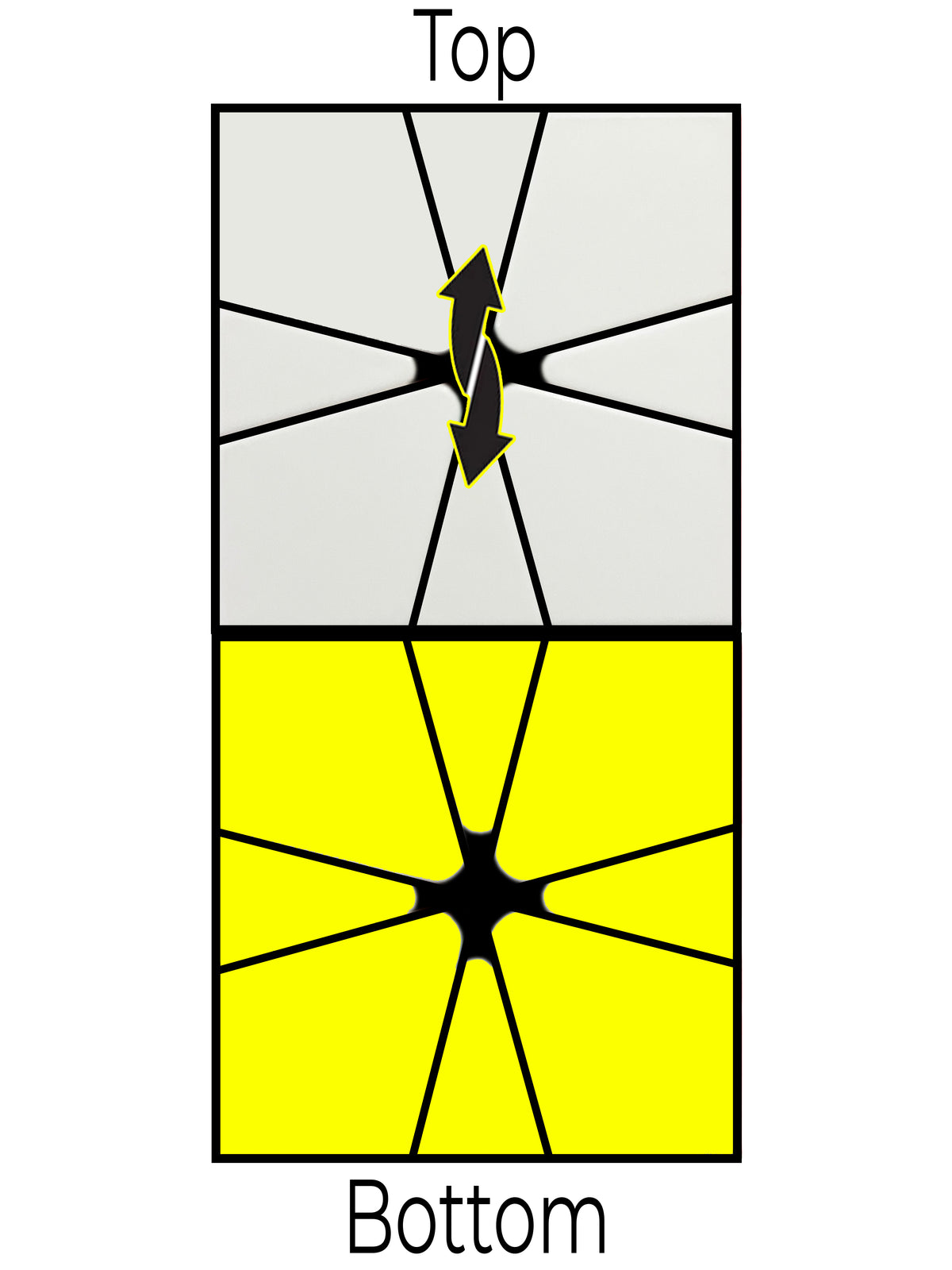Alg 2:       / (3,3) / (1,0) / (-2,-2) / (2,0) / (2,2) / (-1,0) / (-3,-3) / (-2,0) / (3,3) / (3,0) / (-1,-1) / (-3,0 ) / (1,1) / (-4,-3)

Note: Be careful, this is quite a long alg. Take your time if you are a beginner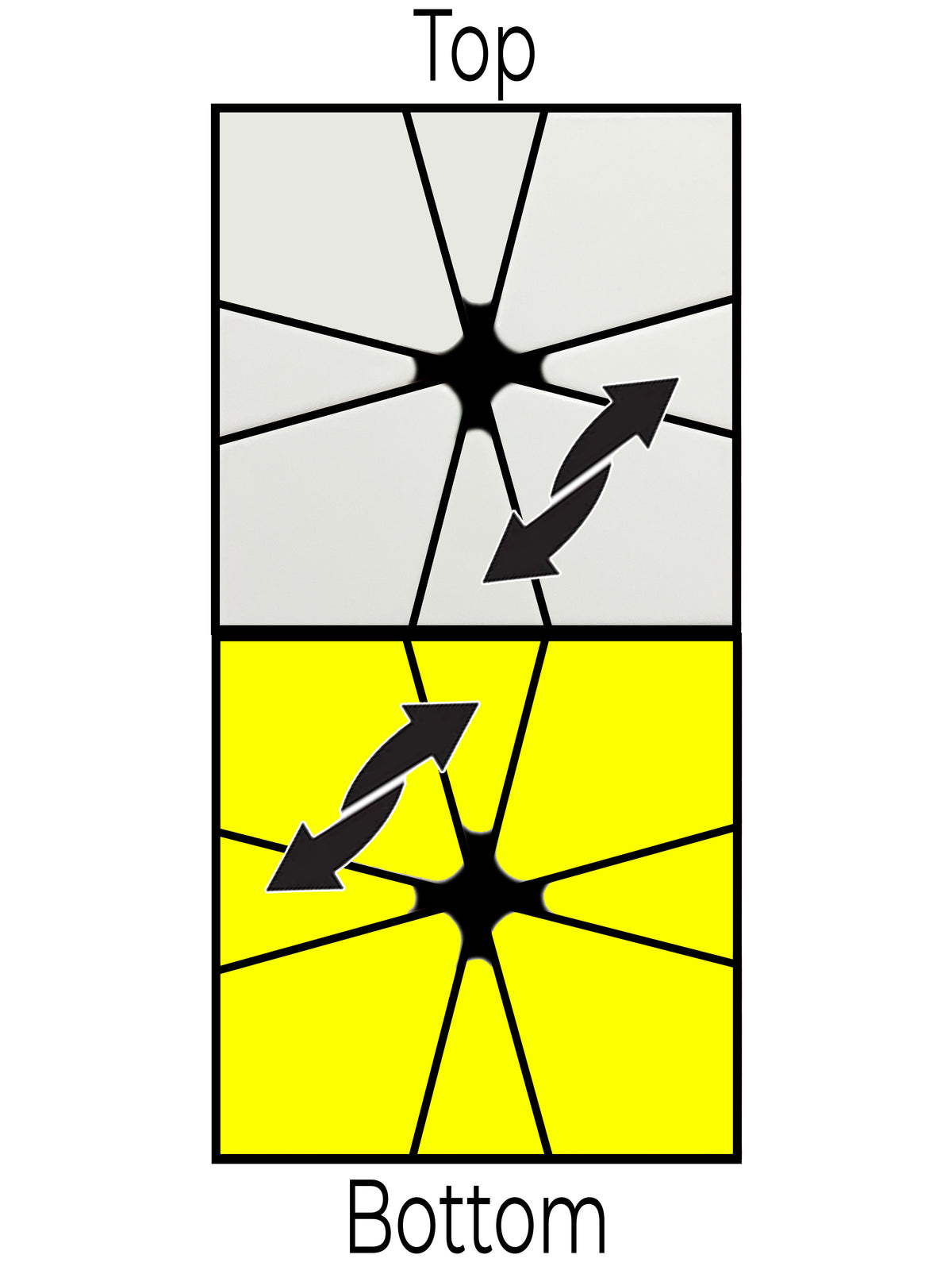Alg 3:       (1,0) / (0,3) / (-1,-1) / (1,-2) / (-1,0)

Tip: (In both of the algs below) between every bottom layer move you are just moving the top layer 1 or -1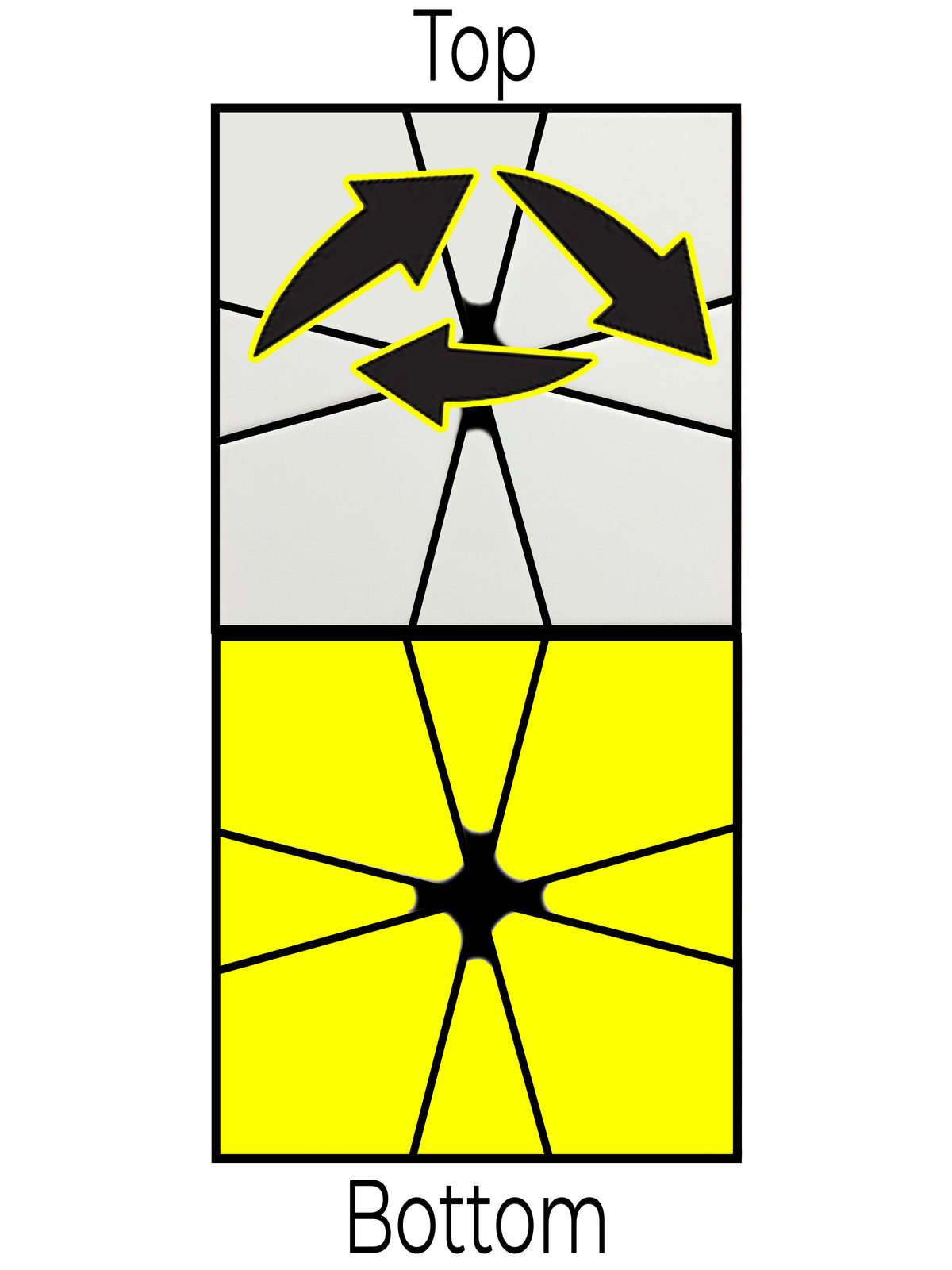Alg 4:       / (3,0) / (1,0) / (0,-3) / (-1,0) / (-3,0) / (1,0) / (0,3) / (-1,0)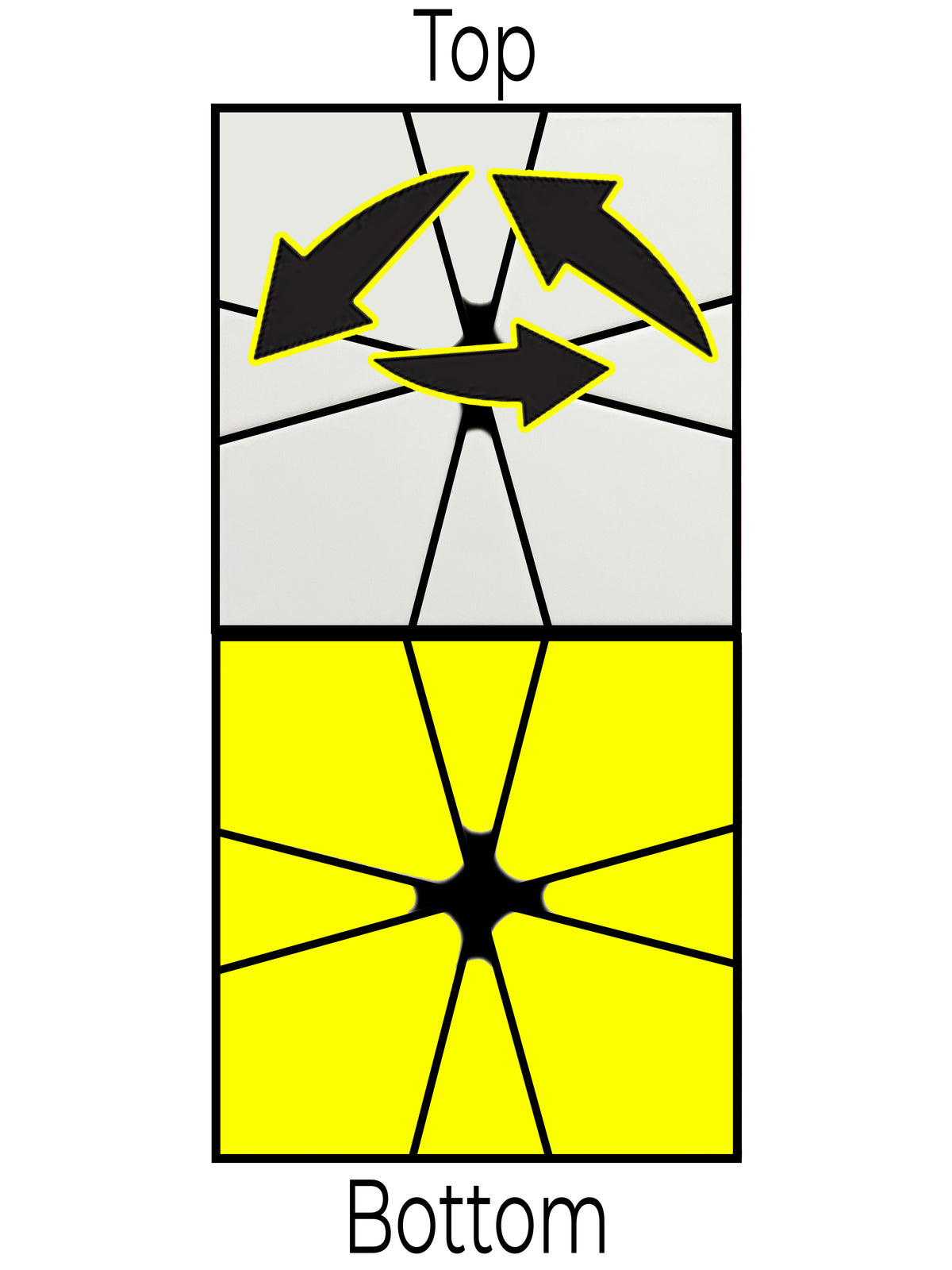Alg 5:      (1,0) / (0,-3) / (-1,0) / (3,0) / (1,0) / (0,3) / (-1,0) / (-3,0) /

Tip: If you need to do any of the moves above but on the bottom layer simply do the algorithm / (6,6) / to switch the top and bottom layers. You can then perform the alg you need to do and switch the layers back with / (6,6) /